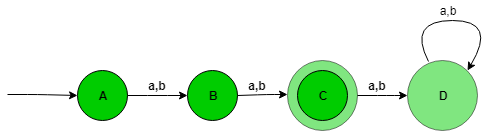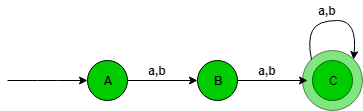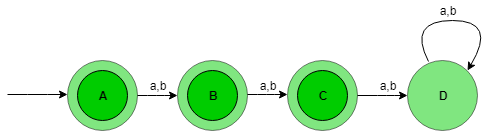# Designing Deterministic Finite Automata (Set 1)

Prerequisite – Designing finite automata
In this article, we will see some designing of Deterministic Finite Automata (DFA).

Problem-1: Construction of a DFA for the set of string over {a, b} such that length of the string |w|=2 i.e, length of the string is exactly 2.

Explanation – The desired language will be like:

`L = {aa, ab, ba, bb} `

The state transition diagram of the language will be like:Here,
State A represent set of all string of length zero (0), state B represent set of all string of length one (1), state C represent set of all string of length two (2). State C is the final state and D is the dead state it is so because after getting any alphabet as input it will not go into final state ever.

```Number of states: n+2
Where n is |w|=n ```

The above automata will accept all the strings having the length of the string exactly 2. When the length of the string is 1, then it will go from state A to B. When the length of the string is 2, then it will go from state B to C and when the length of the string is greater than 2, then it will go from state C to D (Dead state) and after it from state D TO D itself.

 `#check string in ` `#in state A ` `def` `checkStateA(n): ` `     `  `    ``#if length of ` `    ``#string is one ` `    ``#print not accepted ` `    ``if``(``len``(n)``=``=``1``): ` `        ``print``(``"string not accepted"``) ` `    ``else``:    ` `        ``#pass string to stateB to ` `        ``#to check further transitions ` `        ``if``(n[``0``]``=``=``'a'` `or` `n[``0``]``=``=``'b'``): ` `            ``stateB(n[``1``:]) ` `             `  `             `  `def` `stateB(n): ` `    ``#here if length  ` `    ``#is not 1 print#string not accepted ` `    ``if``(``len``(n)!``=``1``): ` `        ``print``(``"string not accepted"``) ` `    ``else``: ` `        ``#else pass string  ` `        ``#to state c ` `        ``stateC(n[``1``:]) ` `def` `stateC(n): ` `    ``#here if length  ` `    ``#becomes zero ` `    ``#print accepted ` `    ``#else not accepted ` `    ``if` `(``len``(n)``=``=``0``): ` `        ``print``(``"string accepted"``) ` `    ``else``: ` `        ``print``(``"string not accepted"``) ` `     `  `     `  `#take input     ` `n``=``input``() ` `checkStateA(n) `

Problem-2: Construction of a DFA for the set of string over {a, b} such that length of the string |w|>=2 i.e, length of the string should be at least 2.

Explanation – The desired language will be like:

`L = {aa, ab, ba, bb, aaa, aab, aba, abb........} `

The state transition diagram of the language will be like:Here,
State A represent set of all sting of length zero (0), state B represent set of all sting of length one (1), and state C represent set of all sting of length two (2).

```Number of states: n+1
Where n is |w|>=n ```

The above automata will accept all the strings having the length of the string at least 2. When the length of the string is 1, then it will go from state A to B. When the length of the string is 2, then it will go from state B to C and lastly when the length of the string is greater than 2, then it will go from state C to C itself.

 `#check string in ` `#in state A ` `def` `checkStateA(n): ` `     `  `    ``#if length of ` `    ``#string is one ` `    ``#print not accepted ` `    ``if``(``len``(n)``=``=``1``): ` `        ``print``(``"string not accepted"``) ` `    ``else``:    ` `        ``#pass string to stateB to ` `        ``#to check further transitions ` `        ``if``(n[``0``]``=``=``'a'` `or` `n[``0``]``=``=``'b'``): ` `            ``stateB(n[``1``:]) ` `             `  `             `  `def` `stateB(n): ` `     `  `    ``#here if length  ` `    ``#is less than 1  ` `    ``#printstring not accepted ` `    ``if``(``len``(n)<``1``): ` `        ``print``(``"string not accepted"``) ` `    ``else``: ` `         `  `        ``#else pass string  ` `        ``#to state c ` `        ``stateC(n[``1``:]) ` `         `  `         `  `def` `stateC(n): ` `    ``#here if length of string  ` `    ``#is greater than equal to zero ` `    ``#print accepted ` `    ``#else not accepted ` `    ``if` `(``len``(n)>``=``0``): ` `        ``print``(``"string accepted"``) ` `    ``else``: ` `        ``print``(``"string not accepted"``) ` `     `  `     `  `#take input     ` `n``=``input``() ` `checkStateA(n) `

Problem-3: Construction of a DFA for the set of string over {a, b} such that length of the string |w|<=2 i.e, length of the string is atmost 2.

Explanation – The desired language will be like:

`L = {?, aa, ab, ba, bb} `

The state transition diagram of the language will be like:Here,
State A represent set of all sting of length zero (0), state B represent set of all sting of length one (1), state C represent set of all sting of length two (2), state A, B, C is the final state and D is the dead state it is so because after getting any alphabet as input it will not go into final state ever.

```Number of states: n+2
Where n is |w|<=n ```

The above automata will accept all the strings having the length of the string at most 2. When the length of the string is 1, then it will go from state A to B. When the length of the string is 2, then it will go from state B to C and lastly when the length of the string is greater than 2, then it will go from state C to D (Dead state).

 `#check string in ` `#in state A ` `def` `checkStateA(n): ` `     `  `    ``#if only two transition occurs  ` `    ``#then print string accepted ` `   `  `    ``if``(n[``0``]``=``=``'a'` `or` `n[``0``]``=``=``'b'``): ` `        ``stateB(n[``1``:]) ` `             `  `             `  `def` `stateB(n): ` `     `  `    ``#if length is 0  ` `    ``#print accepted ` `    ``if``(``len``(n)``=``=``0``): ` `        ``print``(``"string accepted"``) ` `    ``else``: ` `        ``stateC(n[``1``:]) ` `         `  `         `  `def` `stateC(n): ` `    ``#if length is 0  ` `    ``#print accepted ` `    ``#else not accepted ` `    ``if` `(``len``(n)``=``=``0``): ` `        ``print``(``"string sccepted"``) ` `    ``else``: ` `        ``print``(``"string not accepted"``) ` `     `  `     `  `#take input     ` `n``=``input``() ` `checkStateA(n) `

Don’t stop now and take your learning to the next level. Learn all the important concepts of Data Structures and Algorithms with the help of the most trusted course: DSA Self Paced. Become industry ready at a student-friendly price.

My Personal Notes arrow_drop_upCheck out this Author's contributed articles.

If you like GeeksforGeeks and would like to contribute, you can also write an article using contribute.geeksforgeeks.org or mail your article to contribute@geeksforgeeks.org. See your article appearing on the GeeksforGeeks main page and help other Geeks.

Please Improve this article if you find anything incorrect by clicking on the "Improve Article" button below.

Article Tags :

6

Please write to us at contribute@geeksforgeeks.org to report any issue with the above content.